Chapter 3 Two-Dimensional Kinematics

# 18 3.3 Vector Addition and Subtraction: Analytical Methods

### Summary

• Understand the rules of vector addition and subtraction using analytical methods.
• Apply analytical methods to determine vertical and horizontal component vectors.
• Apply analytical methods to determine the magnitude and direction of a resultant vector.

Analytical methods of vector addition and subtraction employ geometry and simple trigonometry rather than the ruler and protractor of graphical methods. Part of the graphical technique is retained, because vectors are still represented by arrows for easy visualization. However, analytical methods are more concise, accurate, and precise than graphical methods, which are limited by the accuracy with which a drawing can be made. Analytical methods are limited only by the accuracy and precision with which physical quantities are known.

# Resolving a Vector into Perpendicular Components

Analytical techniques and right triangles go hand-in-hand in physics because (among other things) motions along perpendicular directions are independent. We very often need to separate a vector into perpendicular components. For example, given a vector like$\textbf{A}$in Figure 1, we may wish to find which two perpendicular vectors,$\boldsymbol{\textbf{A}_x}$and$\boldsymbol{\textbf{A}_y}$, add to produce it.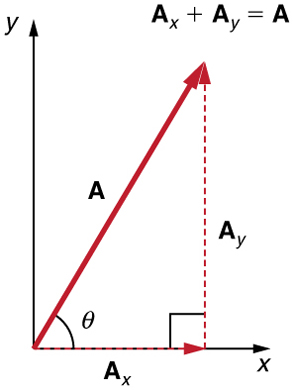$\boldsymbol{\textbf{A}_x}$and$\boldsymbol{\textbf{A}_y}$are defined to be the components of$\textbf{A}$the x- and y-axes. The three vectors$\textbf{A},\:\boldsymbol{\textbf{A}_x},$and$\boldsymbol{\textbf{A}_y}$form a right triangle:

$\boldsymbol{\textbf{A}_x +\textbf{A}_y =\textbf{A}.}$

Note that this relationship between vector components and the resultant vector holds only for vector quantities (which include both magnitude and direction). The relationship does not apply for the magnitudes alone. For example, if$\boldsymbol{\textbf{A}_x=3\textbf{ m}}$east,$\boldsymbol{\textbf{A}_y=4\textbf{ m}}$north, and$\boldsymbol{\textbf{A}=5\textbf{ m}}$north-east, then it is true that the vectors$\boldsymbol{\textbf{A}_x+\textbf{A}_y=\textbf{A}}.$However, it is not true that the sum of the magnitudes of the vectors is also equal. That is,

$\boldsymbol{3\textbf{ m}+4\textbf{ m}\neq 5\textbf{ m}}$

Thus,

$\boldsymbol{\textbf{A}_x+\textbf{A}_y\neq\textbf{A}}$

If the vector$\textbf{A}$is known, then its magnitude$\textbf{A}$and its angle$\boldsymbol{\theta}$(its direction) are known. To find$\boldsymbol{\textbf{A}_x}$and$\boldsymbol{A_y},$ its x- and y-components, we use the following relationships for a right triangle.

$\boldsymbol{\textbf{A}_x=\textbf{A cos}\:\theta}$

and

$\boldsymbol{\textbf{A}_y=\textbf{A sin}\:\theta}.$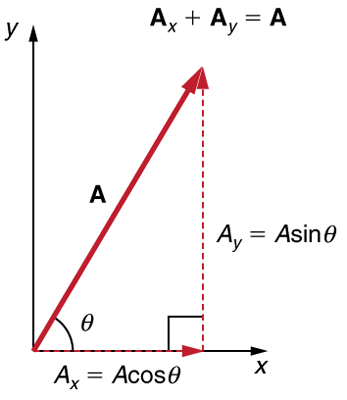Suppose, for example, that$\textbf{A}$is the vector representing the total displacement of the person walking in a city considered in Chapter 3.1 Kinematics in Two Dimensions: An Introduction and Chapter 3.2 Vector Addition and Subtraction: Graphical Methods.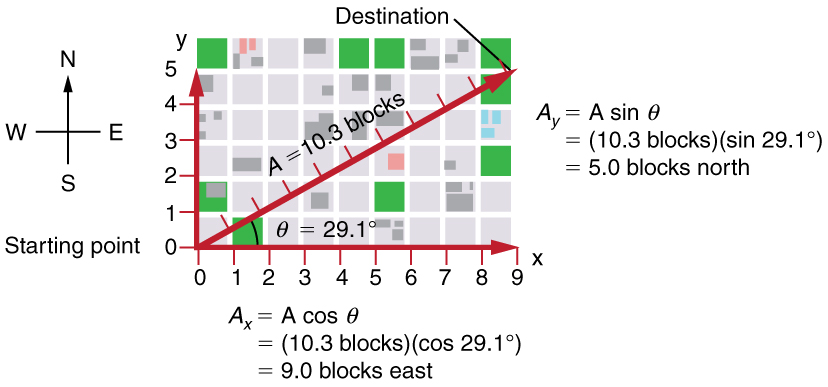Then$\boldsymbol{\textbf{A}=10.3\textbf{ blocks}}$and$\boldsymbol{\theta=29.1^o},$ so that

$\boldsymbol{\textbf{A}_x=\textbf{A cos}\:\theta=(10.3\textbf{ blocks})(\textbf{cos}29.1^o)=9.0\textbf{ blocks}}$
$\boldsymbol{\textbf{A}_y=\textbf{A sin}\:\theta=(10.3\textbf{ blocks})(\textbf{sin}29.1^o)=5.0\textbf{ blocks}.}$

# Calculating a Resultant Vector

If the perpendicular components$\boldsymbol{\textbf{A}_x}$and$\boldsymbol{\textbf{A}_y}$of a vector$\textbf{A}$are known, then$\textbf{A}$can also be found analytically. To find the magnitude$\textbf{A}$and direction$\boldsymbol{\theta}$of a vector from its perpendicular components$\boldsymbol{\textbf{A}_x}$and$\boldsymbol{\textbf{A}_y},$we use the following relationships:

$\boldsymbol{\textbf{A}=\sqrt{A_x\:^2\:+\:A_y\:^2}}$
$\boldsymbol{\theta=\textbf{tan}^{-1}(\textbf{A}_y\:/\:\textbf{A}_x).}$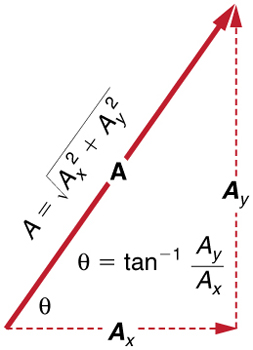Note that the equation$\boldsymbol{\textbf{A}=\sqrt{A_x\:^2\:+\:A_y\:^2}}$is just the Pythagorean theorem relating the legs of a right triangle to the length of the hypotenuse. For example, if$\boldsymbol{\textbf{A}_x}$and$\boldsymbol{\textbf{A}_y}$are 9 and 5 blocks, respectively, then$\boldsymbol{\textbf{A}=\sqrt{9^2\:+\:5^2}=10.3}$blocks, again consistent with the example of the person walking in a city. Finally, the direction is$\boldsymbol{\theta=\textbf{tan}^{-1}(5/9)=29.1^o},$as before.

### DETERMINING VECTORS AND VECTOR COMPONENTS WITH ANALYTICAL METHODS

Equations$\boldsymbol{\textbf{A}_x=\textbf{A cos}\:\theta}$and$\boldsymbol{\textbf{A}_y=\textbf{A sin}\:\theta}$are used to find the perpendicular components of a vector—that is, to go from $\textbf{A}$and$\boldsymbol{\theta}$to$\boldsymbol{\textbf{A}_x}$and$\boldsymbol{\textbf{A}_y}.$Equations$\boldsymbol{\textbf{A}=\sqrt{{\textbf{A}_x}^2\:+\:{\textbf{A}_y}^2}}$and$\boldsymbol{\theta=\textbf{tan}^{-1}(\textbf{A}_y\:/\:\textbf{A}_x)}$are used to find a vector from its perpendicular components—that is, to go from$\boldsymbol{\textbf{A}_x}$and$\boldsymbol{\textbf{A}_y}$to$\textbf{A}$and$\boldsymbol{\theta}.$Both processes are crucial to analytical methods of vector addition and subtraction.

# Adding Vectors Using Analytical Methods

To see how to add vectors using perpendicular components, consider Figure 5, in which the vectors$\textbf{A}$and$\textbf{B}$are added to produce the resultant$\textbf{R}.$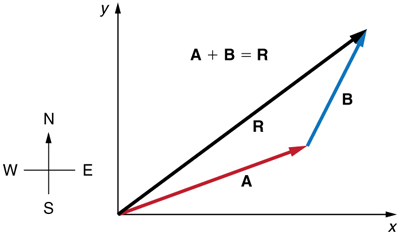If$\textbf{A}$and$\textbf{B}$represent two legs of a walk (two displacements), then$\textbf{R}$is the total displacement. The person taking the walk ends up at the tip of$\textbf{R}.$There are many ways to arrive at the same point. In particular, the person could have walked first in the x-direction and then in the y-direction. Those paths are the x– and y-components of the resultant,$\boldsymbol{\textbf{R}_x}$and$\boldsymbol{\textbf{R}_y}.$If we know$\boldsymbol{\textbf{R}_x}$and$\boldsymbol{\textbf{R}_y},$we can find$\textbf{R}$and$\boldsymbol{\theta}$using the equations$\boldsymbol{\textbf{A}=\sqrt{{\textbf{A}_x}^2\:+\:{\textbf{A}_y}^2}}$and$\boldsymbol{\theta=\textbf{tan}^{-1}(\textbf{A}_y\:/\:\textbf{A}_x)}.$When you use the analytical method of vector addition, you can determine the components or the magnitude and direction of a vector.

Step 1. Identify the x- and y-axes that will be used in the problem. Then, find the components of each vector to be added along the chosen perpendicular axes. Use the equations$\boldsymbol{\textbf{A}_x=\textbf{A cos}\:\theta}$and$\boldsymbol{\textbf{A}_y=\textbf{A sin}\:\theta}$to find the components. In Figure 6, these components are$\boldsymbol{\textbf{A}_x},\boldsymbol{\textbf{A}_x},\boldsymbol{\textbf{B}_x},$and$\boldsymbol{\textbf{B}_y}.$The angles that vectors$\textbf{A}$and$\textbf{B}$make with the x-axis are$\boldsymbol{\theta_{A}}$and$\boldsymbol{\theta_{B}},$respectively.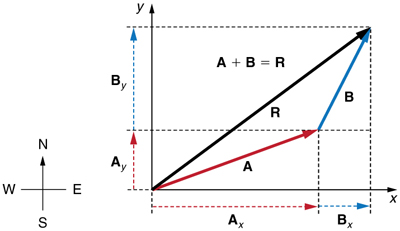Step 2. Find the components of the resultant along each axis by adding the components of the individual vectors along that axis. That is, as shown in Figure 7,

$\boldsymbol{\textbf{R}_x=\textbf{A}_x+\textbf{B}_x}$

and

$\boldsymbol{\textbf{R}_y=\textbf{A}_y+\textbf{B}_y.}$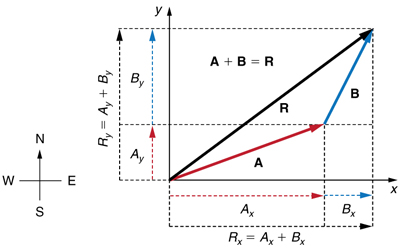Components along the same axis, say the x-axis, are vectors along the same line and, thus, can be added to one another like ordinary numbers. The same is true for components along the y-axis. (For example, a 9-block eastward walk could be taken in two legs, the first 3 blocks east and the second 6 blocks east, for a total of 9, because they are along the same direction.) So resolving vectors into components along common axes makes it easier to add them. Now that the components of$\textbf{R}$are known, its magnitude and direction can be found.

Step 3. To get the magnitude$\textbf{R}$of the resultant, use the Pythagorean theorem:

$\boldsymbol{\textbf{R}=\sqrt{{\textbf{R}_x}^2\:+\:\{textbf{R}_y}^2}.}$

Step 4. To get the direction of the resultant:

$\boldsymbol{\theta=\textbf{tan}^{-1}(\textbf{R}_y\:/\:\textbf{R}_x).}$

The following example illustrates this technique for adding vectors using perpendicular components.

### Example 1: Adding Vectors Using Analytical Methods

Add the vector$\textbf{A}$to the vector$\textbf{B}$shown in Figure 8, using perpendicular components along the x– and y-axes. The x– and y-axes are along the east–west and north–south directions, respectively. Vector$\textbf{A}$represents the first leg of a walk in which a person walks$\boldsymbol{53.0\textbf{ m}}$in a direction$\boldsymbol{20.0^o}$north of east. Vector$\textbf{B}$represents the second leg, a displacement of$\boldsymbol{34.0\textbf{ min}}$a direction$\boldsymbol{63.0^o}$north of east.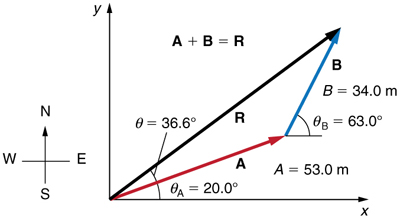Strategy

The components of$\textbf{A}$and$\textbf{B}$along the x– and y-axes represent walking due east and due north to get to the same ending point. Once found, they are combined to produce the resultant.

Solution

Following the method outlined above, we first find the components of$\textbf{A}$and$\textbf{B}$along the x– and y-axes. Note that$\boldsymbol{\textbf{A}=53.0\textbf{ m}},\boldsymbol{\theta_A=20.0^o},\boldsymbol{\textbf{B}=34.0\textbf{ m}},$and$\boldsymbol{\theta_B=63.0^o}.$ We find the x-components by using$\boldsymbol{\textbf{A}_x=\textbf{A cos}\:\theta},$which gives

$\boldsymbol{\textbf{A}_x=\textbf{A cos}\:\theta_A=(53.0\textbf{ m})(\textbf{cos} 20.0^o)}$
$\boldsymbol{=(53.0\textbf{ m})(0.940)=49.8\textbf{ m}}\:\:\:\:\:$

and

$\boldsymbol{\textbf{B}_x=\textbf{B cos}\:\theta_B=(34.0\textbf{ m})(\textbf{cos} 63.0^o)}$
$\boldsymbol{=(34.0\textbf{ m})(0.454)=15.4\textbf{ m}.}\:\:\:\:\:$

Similarly, the y-components are found using$\boldsymbol{\textbf{A}_y=\textbf{A sin}\:\theta}:$

$\boldsymbol{\textbf{A}_y=\textbf{A sin}\:\theta_A=(53.0\textbf{ m})(\textbf{sin} 20.0^o)}$
$\boldsymbol{=(53.0\textbf{ m})(0.342)=18.1\textbf{ m}}\:\:\:\:\:$

and

$\boldsymbol{\textbf{B}_y=\textbf{B sin}\:\theta_B=(34.0\textbf{ m})(\textbf{sin} 63.0^o)}$
$\boldsymbol{=(34.0\textbf{ m})(0.891)=30.3\textbf{ m}.}\:\:\:\:\:$

The x– and y-components of the resultant are thus

$\boldsymbol{\textbf{R}_x=\textbf{A}_x\:+\:\textbf{B}_x=49.8\textbf{ m}\:+\:15.4\textbf{ m}=65.2\textbf{ m}}$

and

$\boldsymbol{\textbf{R}_y=\textbf{A}_y\:+\:\textbf{B}_y=18.1\textbf{ m}\:+\:30.3\textbf{ m}=48.4\textbf{ m}.}$

Now we can find the magnitude of the resultant by using the Pythagorean theorem:

$\boldsymbol{\textbf{R}=\sqrt{\textbf{R}_x^2+\textbf{R}_y^2}=\sqrt{(65.2)^2+(48.4)^2\textbf{ m}}}$

so that

$\boldsymbol{\textbf{R}=81.2\textbf{ m}.}$

Finally, we find the direction of the resultant:

$\boldsymbol{\theta=\textbf{tan}^{-1}(\textbf{R}_y\:/\:\textbf{R}_x)=+\textbf{tan}^{-1}(48.4/65.2).}$

Thus,

$\boldsymbol{\theta=\textbf{tan}^{-1}(0.742)=36.6^o.}$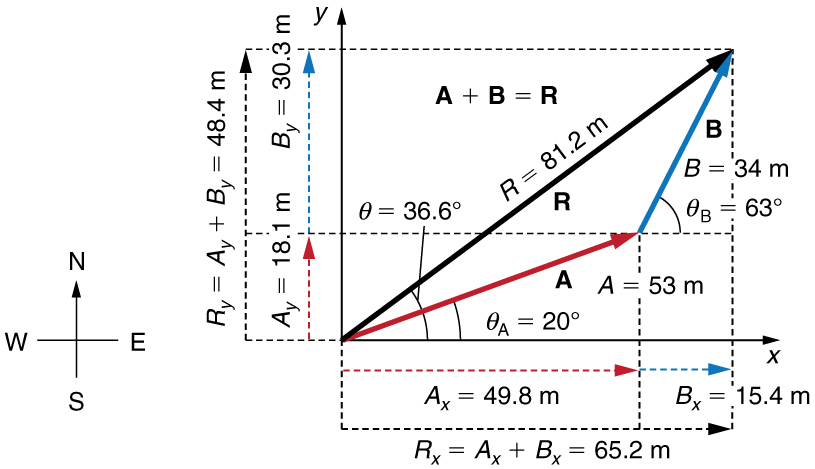Discussion

This example illustrates the addition of vectors using perpendicular components. Vector subtraction using perpendicular components is very similar—it is just the addition of a negative vector.

Subtraction of vectors is accomplished by the addition of a negative vector. That is,$\boldsymbol{\textbf{A}-\textbf{B}\equiv\textbf{A}+(-\textbf{B})}.$Thus, the method for the subtraction of vectors using perpendicular components is identical to that for addition. The components of$\boldsymbol{-\textbf{B}}$are the negatives of the components of$\textbf{B}.$The x– and y-components of the resultant$\boldsymbol{\textbf{A}-\textbf{B}=\textbf{R}}$are thus

$\boldsymbol{\textbf{R}_x=\textbf{A}_x\:+\:(-\textbf{B}_x)}$

and

$\boldsymbol{\textbf{R}_y=\textbf{A}_y\:+\:(-\textbf{B}_y)}$

and the rest of the method outlined above is identical to that for addition. (See Figure 10.)

Analyzing vectors using perpendicular components is very useful in many areas of physics, because perpendicular quantities are often independent of one another. The next module, Chapter 3.4 Projectile Motion, is one of many in which using perpendicular components helps make the picture clear and simplifies the physics.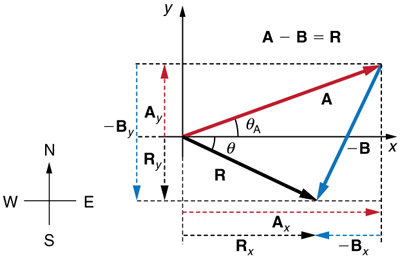Figure 10. The subtraction of the two vectors shown in Figure 5. The components of -B are the negatives of the components of B. The method of subtraction is the same as that for addition.

### PHET EXPLORATIONS: VECTOR ADDITION

Learn how to add vectors. Drag vectors onto a graph, change their length and angle, and sum them together. The magnitude, angle, and components of each vector can be displayed in several formats.Figure 11. Vector Addition

# Summary

• The analytical method of vector addition and subtraction involves using the Pythagorean theorem and trigonometric identities to determine the magnitude and direction of a resultant vector.
• The steps to add vectors$\textbf{A}$and$\textbf{B}$using the analytical method are as follows:

Step 1: Determine the coordinate system for the vectors. Then, determine the horizontal and vertical components of each vector using the equations

$\boldsymbol{\textbf{A}_x=\textbf{A cos}\:\theta}$
$\boldsymbol{\textbf{B}_x=\textbf{B cos}\:\theta}$

and

$\boldsymbol{\textbf{A}_y=\textbf{A sin}\:\theta}$
$\boldsymbol{\textbf{B}_y=\textbf{B sin}\:\theta}.$

Step 2: Add the horizontal and vertical components of each vector to determine the components$\boldsymbol{\textbf{R}_x}$and$\boldsymbol{\textbf{R}_y}$of the resultant vector,$\textbf{R}:$

$\boldsymbol{\textbf{R}_x=\textbf{A}_x+\textbf{B}_x}$

and

$\boldsymbol{\textbf{R}_y=\textbf{A}_y+\textbf{B}_y}.$

Step 3: Use the Pythagorean theorem to determine the magnitude,$\boldsymbol{R},$of the resultant vector$\textbf{R}:$

$\boldsymbol{\textbf{R}=\sqrt{{\textbf{R}_x}^2\:+\:{\textbf{R}_y}^2}.}$

Step 4: Use a trigonometric identity to determine the direction,$\boldsymbol{\theta},$of$\textbf{R}:$

$\boldsymbol{\theta=\textbf{tan}^{-1}(\textbf{R}_y\:/\:\textbf{R}_x).}$

### Conceptual Questions

1: Suppose you add two vectors$\textbf{A}$and$\textbf{B}.$What relative direction between them produces the resultant with the greatest magnitude? What is the maximum magnitude? What relative direction between them produces the resultant with the smallest magnitude? What is the minimum magnitude?

2: Give an example of a nonzero vector that has a component of zero.

3: Explain why a vector cannot have a component greater than its own magnitude.

4: If the vectors$\textbf{A}$and$\textbf{B}$are perpendicular, what is the component of$\textbf{A}$along the direction of$\textbf{B}?$What is the component of$\textbf{B}$along the direction of$\textbf{A}?$

### Problems & Exercises

1: Find the following for path C in Figure 12: (a) the total distance traveled and (b) the magnitude and direction of the displacement from start to finish. In this part of the problem, explicitly show how you follow the steps of the analytical method of vector addition.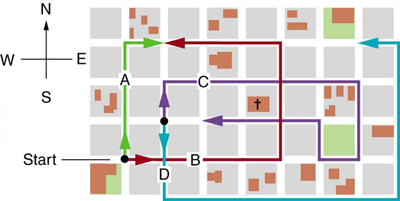2: Find the following for path D in Figure 12: (a) the total distance traveled and (b) the magnitude and direction of the displacement from start to finish. In this part of the problem, explicitly show how you follow the steps of the analytical method of vector addition.

3: Find the north and east components of the displacement from San Francisco to Sacramento shown in Figure 13.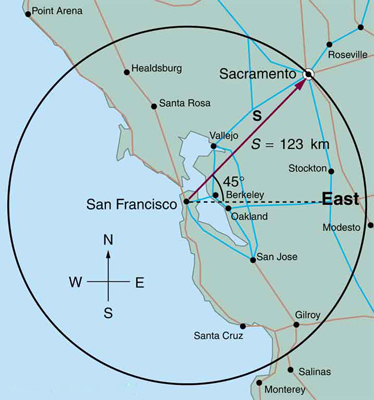Figure 13.

4: Solve the following problem using analytical techniques: Suppose you walk 18.0 m straight west and then 25.0 m straight north. How far are you from your starting point, and what is the compass direction of a line connecting your starting point to your final position? (If you represent the two legs of the walk as vector displacements$\textbf{A}$and$\textbf{B},$as in Figure 14, then this problem asks you to find their sum$\boldsymbol{\textbf{R}=\textbf{A}+\textbf{B}}.)$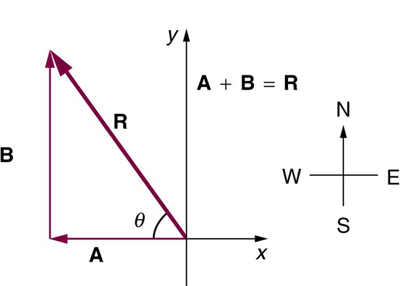Note that you can also solve this graphically. Discuss why the analytical technique for solving this problem is potentially more accurate than the graphical technique.

5: Repeat Exercise 4 using analytical techniques, but reverse the order of the two legs of the walk and show that you get the same final result. (This problem shows that adding them in reverse order gives the same result—that is,$\boldsymbol{\textbf{B}+\textbf{A} =\textbf{A}+\textbf{B}}.)$Discuss how taking another path to reach the same point might help to overcome an obstacle blocking you other path.

6: You drive$\boldsymbol{7.50\textbf{ km}}$in a straight line in a direction$\boldsymbol{15^o}$east of north. (a) Find the distances you would have to drive straight east and then straight north to arrive at the same point. (This determination is equivalent to find the components of the displacement along the east and north directions.) (b) Show that you still arrive at the same point if the east and north legs are reversed in order.

7: Do Exercise 4 again using analytical techniques and change the second leg of the walk to$\boldsymbol{25.0\textbf{ m}}$straight south. (This is equivalent to subtracting$\textbf{B}$from$\textbf{A}$ —that is, finding$\boldsymbol{\textbf{R}^{\prime}=\textbf{A} -\textbf{B}})$(b) Repeat again, but now you first walk$\boldsymbol{25.0\textbf{ m}}$north and then$\boldsymbol{18.0\textbf{ m}}$east. (This is equivalent to subtract$\textbf{A}$from$\textbf{B}$ —that is, to find$\boldsymbol{\textbf{A}=\textbf{B}+\textbf{C}}.$ Is that consistent with your result?)

8: A new landowner has a triangular piece of flat land she wishes to fence. Starting at the west corner, she measures the first side to be 80.0 m long and the next to be 105 m. These sides are represented as displacement vectors$\textbf{A}$from$\textbf{B}$in Figure 15. She then correctly calculates the length and orientation of the third side$\textbf{C}.$What is her result?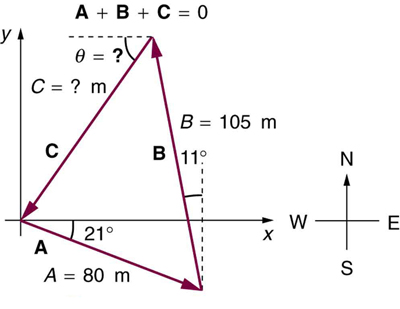Figure 15.

9: You fly$\boldsymbol{32.0\textbf{ km}}$in a straight line in still air in the direction$\boldsymbol{35.0^o}$south of west. (a) Find the distances you would have to fly straight south and then straight west to arrive at the same point. (This determination is equivalent to finding the components of the displacement along the south and west directions.) (b) Find the distances you would have to fly first in a direction$\boldsymbol{45.0^o}$south of west and then in a direction$\boldsymbol{45.0^o}$west of north. These are the components of the displacement along a different set of axes—one rotated$\boldsymbol{45^o}.$

10: A farmer wants to fence off his four-sided plot of flat land. He measures the first three sides, shown as$\textbf{A},\textbf{B},$and$\textbf{C}$in Figure 16, and then correctly calculates the length and orientation of the fourth side$\textbf{D}.$
What is his result?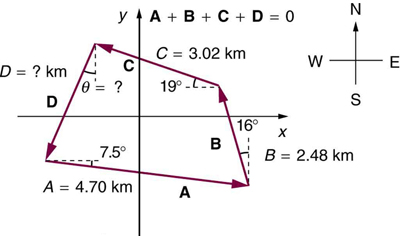Figure 16.

11: In an attempt to escape his island, Gilligan builds a raft and sets to sea. The wind shifts a great deal during the day, and he is blown along the following straight lines:$\boldsymbol{2.50\textbf{ km}\:45.0^o}$north of west; then$\boldsymbol{4.70\textbf{ km}\:60.0^o}$south of east; then$\boldsymbol{1.30\textbf{ km}\:25.0^o}$south of west; then$\boldsymbol{5.10\textbf{ km}}$straight east; then$\boldsymbol{1.70\textbf{ km}\:5.00^o}$east of north; then$\boldsymbol{7.20\textbf{ km}\:55.0^o}$south of west; and finally$\boldsymbol{2.80\textbf{ km}\:10.0^o}$north of east. What is his final position relative to the island?

12: Suppose a pilot flies$\boldsymbol{40.0\textbf{ km}}$in a direction$\boldsymbol{60^o}$north of east and then flies$\boldsymbol{30.0\textbf{ km}}$in a direction$\boldsymbol{15^o}$north of east as shown in Figure 17. Find her total distance$\textbf{R}$from the starting point and the direction$\boldsymbol{\theta}$of the straight-line path to the final position. Discuss qualitatively how this flight would be altered by a wind from the north and how the effect of the wind would depend on both wind speed and the speed of the plane relative to the air mass.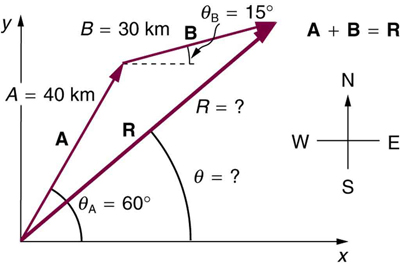Figure 17.

## Glossary

analytical method
the method of determining the magnitude and direction of a resultant vector using the Pythagorean theorem and trigonometric identities

### Solutions

Problems & Exercises

1:

(a) 1.56 km

(b) 120 m east

3:

North-component 87.0 km, east-component 87.0 km

5:

30.8 m, 35.8 west of north

7:

(a)$\boldsymbol{30.8\textbf{ m}},\boldsymbol{54.2^o}$south of west

(b)$\boldsymbol{30.8\textbf{ m}},\boldsymbol{54.2^o}$north of east

9:

(a) 18.4 km south, then 26.2 km west

(b) 31.5 km at$\boldsymbol{45.0^o}$south of west, then 5.56 km at$\boldsymbol{45.0^o}$west of north

11:

$\boldsymbol{7.34\textbf{ km}},\boldsymbol{63.5^o}$south of east

## LicenseCollege Physics: OpenStax by OpenStax is licensed under a Creative Commons Attribution 4.0 International License, except where otherwise noted.